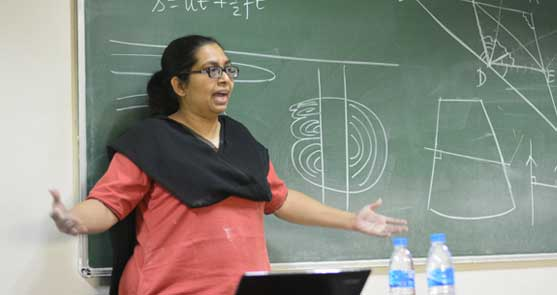## JAIN (Deemed-to-be University) News#### Faculty of Sciences, Jain (Deemed-to-be University) organizes a Session on "Mathematical Modelling and Applications of Differential Equation"As a part of Words of Wisdom series, the Department of Mathematics, Faculty of Sciences, Jain (Deemed-to-be University) organized a session on “Mathematical Modelling and Applications of Differential Equation” on 8 July 2017 by Ms. Swati Sircar, a Senior Lecturer and Resource Person, Azim Premji University.

The speaker elaborated on the various topics of Mathematics which we can observe in our everyday life. The speaker explained the application of Mathematics in sports like cricket and athletics and also the importance of Mathematical equations in the growth of plants.

The speaker pointed out about GeoGebra which is a technique of plotting graphs, finding minima and maxima of the curves. The speaker explained the beauty of Mathematics in Saree folding (can find the power of 2s) and in Champa flower (can find the power of 3s) and discussed various topics like origami, making of airbags, medical sciences, launching of satellites and the Mathematics behind it. The speaker also discussed the topic ‘Doubling time in Exponential Growth’ and ‘Puzzle related to Mathematical Modeling’.

The informative session was concluded by felicitation to the speaker by Dr. Arathi Sudharshan HoD, Mathematics and vote of thanks by Mr. Ramana Raju, Dept. of Mathematics.